## Friday, December 2, 2022

### Momentum: Notes and Such

Physics Index

Where are we going with this? The information on this page connects to standards such as:

• Using experimental evidence and investigations, determine that Newton’s second law of motion defines force as a change in momentum, F = Δp/Δt.

• Develop and apply the impulse-momentum theorem along with scientific and engineering ideas to design, evaluate, and refine a device that minimizes the force on an object during a collision (e.g., helmet, seatbelt, parachute).

•  Assess the validity of the law of conservation of linear momentum (p=mv) by planning and constructing a controlled scientific investigation involving two objects moving in one-dimension.

Momentum: Notes and Such
(Wow! Now, that's a fancy title!)

What is momentum? Well… It's not a force… And it's not energy… Basically, it's a thing that helps us understand motion. And collisions.

(You gotta do better than that!)

A more formal definition would say that momentum is the quantity of motion, the product of an object's mass and its velocity, and it can be quantized as

p = mv

where is p is momentum, m is mass, and v is velocity.

Momentum is… boring… unless something changes. Just watching a football player run at a constant velocity isn't very interesting.

Where momentum becomes interesting is when something happens. Momentum concepts can then help us describe it.

The first application is when momentum changes… So, you got a thing with some momentum. Then something happens and there is less mass. Or more. Or the velocity changes.

This is sort of an abstract concept that helps us illustrate that (this is important!)…

within any closed system, momentum is conserved. Unless something different is introduced to the system, momentum is a constant.

Hence, within a closed system, any changes are limited to within that system, so the momentum can be thought of as having a first and second state (and 3rd, 4th, etc… if you so desired). So, we can formulize that to say that

p1 = p2

And since p = mv, then it follows that

m1v1 = m2v2

This will lead to questions where WITHIN THE CLOSED SYSTEM the mass or velocity will change (by means that somehow do not violate the "closed system" concept).

A far more realistic application is one in which something affects the moving object and thus causes a change to the momentum. This gives rise questions that examine the m1v1 = m2v2 in momentum from a first condition to a second (and third, fourth, fifth… if so desired).

This can be represented as…

∆p = p2 - p1
∆p =  m2v2  -  m1v1       <--- plug in                (eq. 1

Frequently, this will be seen when the velocity of an object changes. Since it is the same object

m1 = m2

So the formula can be represented as:

∆p = m(v2 - v1)                         (eq. 2

The astute observer will recognize that

v2 - v1 = ∆v

Leading to the fancified version of equation 2 shown below

∆p = m∆v                         (eq. 3

A less frequent possibility is where mass changes (or when both mass and velocity change). Equation 1 can be a starting point for those situations.

IMPORTANT NOTE
Momentum is the product of velocity and mass, and velocity has a direction, so therefore, momentum has a direction (it is a vector). You will have to attend to the positive and negative values as directions.

Further, (LIKE ALL VECTORS), momentum need not occur on a single axis. Vectors can be deconstructed into orthogonal components, then all of the componentes can be recombined into resultant vectors.

### Force and Momentum

Another important consideration of momentum is the forces involved in creating momentum change.

This concept assumes that, for some period of time, a force of some magnitude and direction act on an object causing a change in velocity which causes a change in momentum. Let's have a look!

∆p = m∆v         (Eq. 1

Now, what causes velocity to change? Acceleration!

∆v = a∆t        (Eq. 2

Now, how is force related to acceleration?

F = ma
a = F/m         (Eq. 3

Plug a from Eq. 3 into Eq. 2 we get

∆v = (F/m)∆t        (Eq. 4

Now, plug Eq. 4 into Eq. 1 to get…

∆p = m  F/m  ∆t
∆p = F∆t      <--- Cancel the m in above               (Eq. 5

One more version…  Substitute Eq. 1 into Eq. 5:

m∆v = F∆t            (Eq. 6

Different situations will call for the use of different equations, so look at what is given and pick accordingly.

Up to this point, we have examined momentum only from the perspective of a single object. However, the interaction of more than one object leads many interesting outcomes.

This is usually considered collisions

### Collisions

There are two types of collisions.

In an elastic collision, two things collide and bounce off each other, going off separately after the collision.

In an inelastic collision, two things collide and stick to each other, going off as a single thing after the collision.

### Collisions (Both)

In a system involving a collision, we look at the two (or more) objects as they interact. We look at them in isolation, generally. That is to say, we only consider how they are interacting with each other.

In this case, we have a closed system in which momentum is conserved. That means that, where case 1 is before the collision and case 2 is after,

pinitial = pfinal            (Eq. 1

Since the total momentum of a system is the momentum of all the objects in it, for any case we can say that

ptot = p1 + p2 + …

### Elastic Collisions

Since momentum for any object is

p = mv

then

ptot = m1v1 + m2v2 + … (Eq. 2

If we limit our discussion to two objects, we can roll Equation 2 back into Equation 1 and get

pinitial = pfinal
m1v1i + m2v2i = m1v1f + m2v2f

Woooo… That's a lot of subscripts!

We can make the formula easier on the eyes if we do this…

let u be initial velocity and v be final velocity

Since mass before and after does not change, then:

pinitial = pfinal
m1u1 + m2u2 = m1v1 + m2v           (Eq. 3

Equation 3 is easy to solve if you have seven of the eight values given.

Often, though, you need to find one of the final velocities, but know the value of neither. Yikes!

Through a fairly tedious algebra process, you can arrive at the following formulas:

v1=(m1-m) / ( m1+m2) • u1    +    2m2 / (m1+m2) • u2              (Eq. 4

v2=(m2-m1) / (m1+m2) • u2  +    (2m1 / (m1+m2) • u1                    (Eq 5

You will see this in different forms, if you do research. You might see the second arranged like this:

v2(2m1 / (m1+m2) • u1   +  (m2-m1) / (m1+m2) • u2                    (Eq 5 alt

It's math! You can rearrange things following the… you know… math rules!

### Inelastic Collisions

Okay, this time, the two things crash and "stick" to each other. As a result, we have 2 initial mass, but ONLY 1 FINAL MASS.

Jump back to Equation 3 above…

pinitial = pfinal
m1u1 + m2u2 = m1v1 + m2v           (Eq. 3

Now, this time, m1 and m2 become stuck to each other. They go off as one thing, with only one mass and only one velocity. So, what we get is…

let u be initial velocity and v be final velocity

m1u1 + m2u2 = (m1+ m2)v            (Eq. 3

I like to think of it as…

m3 = m1 + m2

This makes the the equation

m1u1 + m2u2 = m3v            (Eq. 3 alt

## Wednesday, November 30, 2022

### Buoyancy: Notes and Such

Physics Index

Where are we going with this? The information on this page connects to standards that create understanding of the forces and interactions between objects is important for describing an object’s motion and determining the stability in a system. Students should understand that forces between objects arise from four types of interactions (gravitational, electromagnetism, and strong and weak nuclear interactions) and that some physical systems are more stable than others.

Buoyancy: Notes and Such
(Okay, this should be interesting!)

Recalling that the formula for buoyancy is

Fb  = -ρgV

a little discussion is worthwhile. First off, buoyancy is in the opposite direction of gravity. Hence, the negative sign at the front of the equation.

The rho (ρ) stands for density of the fluid. The g is acceleration due to gravity. The V is the volume of the fluid.

So, in essence, the upward force is equal to the weight of the fluid displaced.

If you displace 10 pounds of water (say you are floating a fireproof box), the upward force is 10 pounds. If you diplace 10 pounds of lava, the upward force is 10 pounds. Naturally, 10 pounds of water has a much higher volume than 10 pounds of lava.

Generally, in physics, we work with kilograms, liters, and Newtons.

When a solid object is placed in/on a fluid, it will either sink or float. If the weight of the fluid displaced before it goes under is greater than the object's weight, it will float. If not, it will sink. So, we can say the force of weight (mg) of a floating object is equal to weight of the displaced fluid on which it is floating.

Fweight = Fbuoyancy
mg = -ρgV

Conveniently, both the fluid and the object are affected by the same gravity, so the above relationship is true regarding mass, as well. The mass of a floating object is equal to the mass of the displaced fluid on which it is floating.

When an object sinks, it still displaces some fluid. Thus, there is an upward force of some amount, but it is less than the force of weight.

If you were to weigh a can of (not diet) soda in the air, then weigh it sunken in water, the weight would be less. It would decrease by an amount equal to the weight of the water displaced. (Plot twist, a lot of diet sodas in a can will float.)

One more thing before we summarize… When the fluid is water, we can enjoy the fact that 1 liter of water weighs nearly, almost exactly 1 kilogram.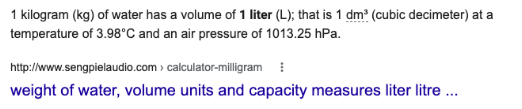Source: https://www.sengpielaudio.com/calculator-milligram.htm

Floating Objects

Objects float because they displace fluid with a weight equal to theirs before they go below the surface of the fluid.
• The weight of the displaced fluid is equal to the weight of the floating object.
• The mass of the displaced fluid is equal to the mass of the floating object.
If the fluid is water, the volume of the water displaced is equal to the mass of the water displaced.

Sunken Objects

Objects sink because they don't displace enough fluid. So…
• The weight of the displaced fluid is less than the weight of the sunken object.
• The volume of the displaced fluid is equal to the volume of the sunken object.

The "underwater" weight of the sunken object is less than the "above-water" weight of the object. It is reduced by an amount equal to the weight of the displaced fluid.

If the fluid is water, the volume of the water displaced is equal to the mass of the water displaced.

## Wednesday, October 5, 2022

### Applied Concepts: Elastic Thing Launcher

Where are we going with this? The information on this page connects projectile motion with conservation of energy where in potential energy is converted to how far a projectile travels.

Applied Concepts: Elastic Thing Launcher
(Okay, this should be interesting!)

Projectile motion is a fundamental aspect of the study of motion in physics. There are many ways to impart velocity at some angle to a projectile.

One way is to attach a "pusher" to some sort of spring or elastic band. Pull it back… BAM!

Think of a slingshot… Now, let the cup ride on rails…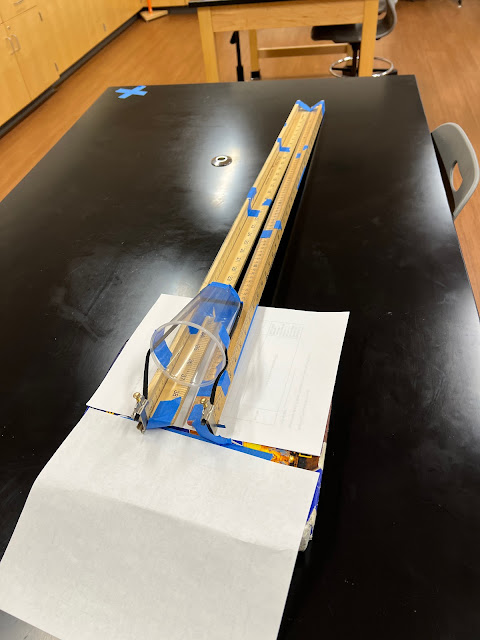The projectile, a squishy ball, rides in the cup that is pulled backand released.

Four quantities are easily measured in this apparatus: The mass of the projectile, the angle of the "thrower," how far back the elastic band was stretched, and how far (distance) that the projectile travels.

From those, everything else can be calculated! Really…

An interesting exercise would be to reverse math back to the coefficient of elasticity in the elastic band (or spring).

Says who?

Stretching the elastic band stores potential energy in the band. And, of course, there is a formula for that!

PE = 1/2kd2

where PE is elastic potential energy, k is the elastic constant, a function of the material, and d is the distance that the spring / band is stretched.

Often you will see this as

PE = 1/2k∆d2

where ∆d is the stretch. Of course, other notations exist! Of course.

Okay… so… When you release the band, most of the energy stored is converted to kinetic energy. While some of that energy will be in the "pusher" (cup in image above) if the mass of the cup is small compared to the projectile, then it's probably okay to ignore it. An even smaller amount will go into friction as the cup rubs on the rails.

Suppose the mass of the "pusher" is 7 grams and the mass of the projectile is 14 grams… Hmm… I'm betting someone weighed something! Then we can say that 2/3 of the potential energy goes into the projectile and 1/3 goes into the "pusher."

So, what now?

Measuring the angle of the rails and the distance that the projectile travels leads to being able to find the initial velocity of the projectile:

_____________

SUMMARY

So, if you are given theta and the velocity, here's a checklist sort of process…
• #1 Draw the diagram and label everything.

• #2 Find the component velocities in the x and y directions:
v • cosθ = vx
v • sinθ = vy

• #3 Find the time up using vy and acceleration due to gravity (probably 9.81 m/s/s)
tup = v / 9.81

• #4 Find the total time where…
t = tup + tdown

and

tup = tdown

so

t = 2•tup

• #5 Use the distance equation to find the horizontal displacement:

d= vx•t
where vx was found in step 2 and was found in step 4.

• #6 BONUS: Find the maximum vertical displacement:

dy = vytup + 1/2atup2
where a = -9.81 m/s/s,  vy was found in step 2 and tup was found in step 3.

_____________
GIANT MESS But actually COOL

d= v• t
dv • cosθ • t
dv • cosθ • 2 • tup
dv • cosθ • 2 • v / 9.81
dv • cosθ • 2 • v • sinθ / 9.81
d= 2 • v2 • cosθ • sinθ / 9.81
wait for the magic!
d= 2 • v2 • 1/2 • sin2θ / 9.81
d=  v2 • sin2θ / 9.81

Say you wanted to find v for some distance x?

9.81 • d=  v2 • sin2θ

(9.81 • d) / sin2θ =  v2

√ (9.81 • dx ) / sin2θ   = v

_____________

Okay, so… wow! Is this why we take math classes?

Thus, from the distance and and angle we get the velocity.

And since the potential energy was converted to kinetic energy…

Whereas

EK = 1/2mv2

and whereas

EK ≈ EP

We can backtrack to find the elasticity.

Considering the materials used, we earlier decided that 2/3 of the potential energy was transferred to the projectile. Thus, in this specific case, and recalling that

PE = 1/2kd2

EK = 2/3 EP

We can state that:

1/2mv2 = 2/3 (1/2kd2)

Thus, after finding v, solving for k becomes an exercise easily completed by the reader.

## Monday, September 19, 2022

### Rounding Numbers (is easy)

Where are we going with this? Rounding off numbers in math is very important to accurately communicate science information

Rounding Numbers (is easy)
(Round numbers? Like zero, six, nine, and eight? Is 3 a round number or a crooked number? And what about two?)

The idea of rounding numbers is to express a long decimal number more concisely. For this discussion, the whole principle of significant figures is going to be passed over. We are going to just be looking at the rounding process.

### Step 1

Figure out how many decimal places you need. This could be dictated by directions in a problem or proper use of significant figures.

In the examples that follow you will be rounding to ONE decimal place.

### Step 2

Look at your number. Find the decimal place. Count over to the digit you are going to round.

Perhaps, as you develop skills, you should underline that number.

EXAMPLE:   1234.7ABxyz

VITAL TO UNDERSTAND!!!!

The only things that can change are:

1. The digit to which you are rounding (underlined above).

2. The digit that comes before it (italics above). There is a 95% chance you will NOT change this.

### Step 3

Look at your digit that comes after the one you are rounding off. In the example it's the green A.

EXAMPLE:   1234.7ABxyz

1234.9ABxyz

becomes

## Friday, August 12, 2022

### Batteries in series and parallel

Physics Index

Where are we going with this? The information on this page introduces how batteries work in series and in parallel.

Batteries in series and parallel
(I don't this will be shocking to very many people!)

Most people are familiar with batteries of some sort. Your wireless mouse and TV remotes might require AA batteries. You probably know your car has a battery. Your cell phone has a battery… so, yeah…

The dry cell battery is a convenient point of reference. You probably will think of a AA, AAA, or D battery. Maybe the C battery.

### Current and Plumbing Analogy? Why not?

Voltage can be thought of as how much pressure is in a circuit. It is somewhat analogous to water in pipes. Higher voltage = higher pressure.

Amperage, however, is not pressure. It is pretty much a measure of the number of electrons coming out of a circuit. Considering the plumbing analogy, it is how many gallons of water are coming out of the pipe at any time.

So, amperage is the AMOUNT of electrons coming out. Voltage is, in this metaphor, kinda like how fast they are coming out or the pressure at which they are coming out.

The math on amperage is fairly intuitive.

If you have 2 hoses that put out 2 gallons per minute, then you are getting 4 gallons per minute in total. If you have 3 hoses at 2 gallons per minute, you are getting 6.

When batteries are connected in parallel, the amperage produced adds up.

Two batteries producing 2 amps each would put out a total of 4 amps. Three of them would produce 6 amps. Etc.

Voltage in parallel would still come out at the same pressure.

Connecting batteries in series, though, is like having one of the hoses filling up the tank from which a second hose is being filled. The second hose can only put out a set amount of water per minute. The first hose just keeps refilling the second hose’s tank. Water comes out until both tanks are empty.

With regard to voltage,  more to come

Back to batteries…

When connected in series, the amperage for any given period of time is unchanged (but the total amount of electrical current is increased). You will get the same amperage for a longer period of time.

In series…

Amp(total) = Amps

In parallel…

Amps(total) = Amps(1) + Amps(2) + …

 Batteries in Series and Parallel(Batteries in the circuit are of same amperage and voltage) Series Parallel Amps A(tot) = A1 = A2 =… A(tot) = A1 + A2 +… Volts V(tot) = V1 + V2 +… V(tot) = V1 = V2 =…

## Monday, August 1, 2022

### Moon Phases and Tides Overview

Physics Index

Where are we going with this? The information on this page introduces explores how the moon affects tides.

Moon Phases and Tides Overview

Let's Just Look at the Moon for a Moment…

Whereas it takes approximately 24 hours for the earth to rotate under the moon, the moon will appear to be directly overhead close to 6 hours after it rises, then will set around 12 hour after it rises. It will then be directly opposite around 6 hours after it sets. (For the purposes of this discussion, we are going to just round off to 24 hours for the period of a day.)

As a convenient way to describe the moons position, we can adopt the term "directly overhead" to mean that it is on the same side of earth as and well into the sky above the observer. (Officially, this is the zenith). It is directly up there! Directly "above" the viewer1.  (There's a footnote. Read it.)

The "horizons" would be to the east and west of the observer.

Directly underfoot would be the opposite. When the moon is above the opposite side of the planet of the observer, we can call that "underfoot." (Officially, it is called the nadir.)

Wouldn't scientist make up fancy words for all that?

The scientific way of saying that the moon is directly overhead is say it is passing through the meridian.

The meridian is a line that passes through a point directly above any point on earth (zenith) and is at a right angle to a line that is tangential to the curve of the earth at that point and passes through the celestial poles. (There's a footnote. Read it.)

If you go around the curve of the earth by 180°, you arrive at the antimeridian. The antimeridian is the line that passes through a point that "opposite" the zenith (which is called the nadir) andalso passes through the celestial poles. (There's a footnote. Read it.)

Now, The Moon Is ALSO Moving…

Not only is the earth turning under the moon, but the moon is also moving. It orbits the earth in the same direction direction as the earth is rotating. It takes the moon 27 days, 7 hours, and 43 minutes to fully orbit the earth.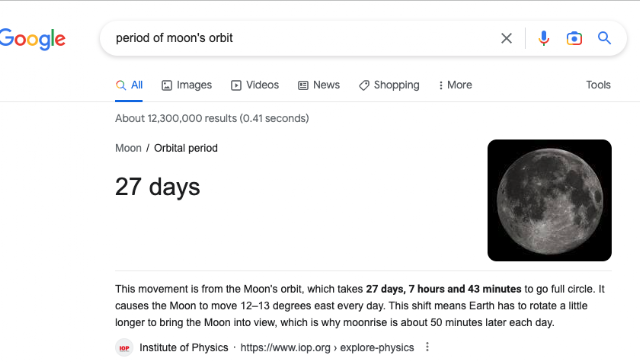Source

The moon's orbit has an effect on when the moon rises and sets and causes the phases of the moon. In the tables below, we can see that moonrise, moonset, and when the moon passes the meridian change from day to day by a similar amount.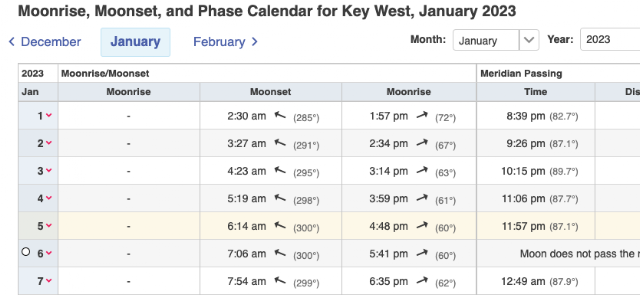Moonrise, moonset, and passing the meridian occur at predictable times each day. The event will occur later each day. But its complex!

The earth is also moving (around the sun). So, the earth rotation changes when sunrise and sunset is (longer days in winter and all that). As it moves, its titled rotational axis affects where the moon's orbital plane lines up with the earth.

As an oversimplification, we can say that the lunar events (moonrise, moonset, etc.) happen about fifty minutes later each day.

Sort of. Depending on where you are, and time of year, and… Notice in the Collierville, TN data above that the events are closer to 40 minutes later, because of season and latitude, and… astronomy!

Now, Let's Look At Tides…

In general…

The fundamental cause of the tides is the gravity of the moon, and then to a much less extent, the gravity of the sun (and then much less than that, the gravity of everything else in the universe).

Whereas the moon is (comparatively) very close to earth, its gravity has the biggest effect.

On earth, ocean tides "run with the moon." To oversimplify matters, a high tide will occur around when the moon is directly overhead or directly underfoot.

Keep in mind that more than just the moon affects tides. Weather and the land masses change the magnitude of the effect the moon has the tidal levels. The timing, however, closely follows the moon's position as the earth rotates under it. (The moon also moves around the earth, which has an impact on timing, too.)

…but predicting the timing of tides is not that simple.

The earth is turning and the moon is moving, so the tides and the moon do not perfectly align. Further the geology of the earth has a big influence on how high a tide will be and how long it will last.

For examples, look at the charts and tables in the footnote section.

The tides are affected by the very nature of the oceans being a liquid. The water is going to respond to the pull of gravity, both the moon's and the earth's. PLUS, the spinning earth acts on the water.

Think about this… When the moon is directly above the center of a North America, it is pulling the water of the Atlantic Ocean toward it with some force. At the same time, the earth spinning, causing the water to move in the direction of the spin. Meanwhile, the earth's gravity is trying to pull the water back into the ocean basin.

For some time, the moon's pull wins out, pulling the ocean toward it, up the beaches on the east coast and creating high tides.

But eventually, the moon's pull is overcome by Earth's pull, so the water slides back out to the middle of the Atlantic. Then, around comes the moon again the next earth rotation, first pulling the water towards Africa and Europe, then pulling it back toward North America.

Though this seems relatively straightforward it actually is more complicated. The complexities of geology and fluid dynamics alter when high and low tide actually occur.

"If Earth was a true sphere covered by an ocean of constant depth, then it would be true that a high tide event would occur at the location with the moon overhead. The tidal "bulge" would move around the Earth with the moon, but [the earth is far from being a perfect sphere]" Source, 2022-01).

The tidal bulge is actually chasing the moon as viewed from earth (if we ignore the continents). There is some confusion in how this is described by various sources. Some will say the tidal bulge comes after the moon passes. Some say it comes ahead of the moon passing (e.g. NASA). One attempt to sort this out states that:

"The bulge moves ahead of the Moon viewed from space, but the Moon apparently moves ahead of the bulge, viewed from the surface of the Earth. They're saying the same thing but from two different perspectives" (Source, 2023-08).

Back to that NASA claim… The call it "Tidal Lag." So… that…

Let's see if we can sort this out a bit…

We have an irregular sphere-like planet spinning and orbiting the sun. We have the moon orbiting the earth as it orbits the sun…

In the image, the earth is spinning counter counter-clockwise. The spinning earth affects the water by pushing it in the direction of the spin. Meanwhile, the moon is pulling the water towards it.

Now, an observer on earth (red dot) will pass under the moon, then into the high tide (tidal bulge).

The net effect of all this is that, from earth, a person will look up and see the moon pass, then ABOUT AN HOUR LATER (to ignore the geography), the person will notice the high tide.

Observed from outer space, the bulge is (because the spinning earth is pushing it) ahead of the moon.

Weather also has a factor in tides. Hurricane storm-surge is an extreme example of this, but wind of any sort will have an effect bodies of water.

So, to wrap up the idea of tides, it is challenging to develop rules. HOWEVERthe "hard and fast" rule is that the moon pulls the water toward it as it moves around the earth and as the earth rotates under it.

Beyond that, to say the EXACT timing of tides is complicated. But Timing of high and low tide will be directly related to lunar events (moonrise, moonset, passing the meridian and passing the antimeridian).

• There will be two high tides daily, roughly connected to when the moon is directly overhead and directly underfoot (opposite). The high tides will occur sorta-kinda 12 hours apart.

• There will be two low tides daily, coming when the moon is on the horizons. Low tides will be around 6 hours after high tides.

## Phases and Tides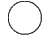Full phase occurs when the Moon is on the opposite side of the Earth from the Sun and we see only the illuminated side. On that day it rises as the Sun is setting and sets and the Sun is rising. It is visible all night long. High tide is, therefore, associated with midnight and noon.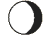Waning Gibbous phase occurs when the Moon is mostly lit and the illuminated portion is egg-shaped (gibbous) with the western edge shaded. The amount of illuminated area visible is decreasing from one day to the next which is what is meant by "waning".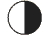Third Quarter phase occurs when the eastern half of the Moon is illuminated. On that day it rises at midnight and sets at noon. It will be directly overhead six hours after it rises (sunrise)  High tide is therefore associated with sunrise and sunset.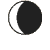Waning Crescent phase occurs when the eastern edge of the Moon is lit but most of the visible surface is dark. The amount of illumination is decreasing from day to day which is what is meant by "waning." During this time the illuminated portion of the Moon looks like the letter "C".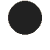New phase occurs when the Sun and Moon are on the same side of the Earth and we see only the dark side. On that day the Sun and Moon rise and set approximately together. The new moon is visible all day long. High tide is, therefore extra high and associated with noon. A second high tide will be associated with midnight.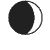Waxing Crescent phase occurs when the western edge of the Moon is lit but most of the surface visible from Earth is dark. The amount of illumination visible is growing from day to day during this phase which is what is meant by "waxing."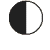First Quarter phase occurs when the western half of the Moon is illuminated so that it looks like the letter "D". On that day the Moon rises at noon and sets at midnight. High tide is, therefore, associated with sunset and sunrise.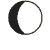Waxing Gibbous phase occurs when the Moon is mostly lit and the illuminated portion is egg-shaped (gibbous) with the eastern edge shaded. The amount of illuminated area visible is increasing from one day to the next which is what is meant by "waxing".

### Key Points:

• Tides on earth are primarily caused by the gravitational pull of the moon. It draws the waters of earth to it (noticeable in oceans and other LARGE bodies of water).

• Whereas the moon is moving as the earth rotates under it, the waters are always "chasing" the moon.

• The magnitude of tidal fluctuation and timing are strongly connected to the physical geography of earth. As the waters of the ocean try to "run with" the moon, they will pile up on land masses.

• At high tide, the level of the ocean is higher. Thus, LESS of a beach will be visible. At low tide, the ocean is lower. Thus MORE of the beach will be visible.

_______________

1Full disclosure AKA, "It's not that simple.": the moon's path sort of wobbles around the equator due to it having an elliptical orbit that is around 5° tilted relative to the equator. Thus, depending on where you are on earth, the moon will be to the north or south of you by some amount, so "overhead" is actually not straight up. So, to say "directly overhead" means that it is perfectly on a north-south line on the same side of earth as the viewer. "Directly underfoot" would mean that it is exactly 180° away from the same line.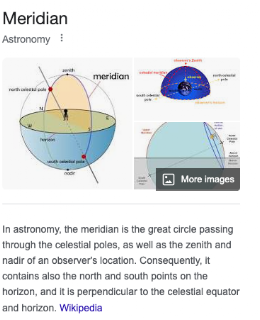https://www.google.com/search?q=astronomy+meridian

That line has an official name

So do a couple of other points…

The zenith is a point directly over the observer's head.

The nadir is a point directly under an observer's feet.

The celestial poles are points directly above the north and south pole (not "magnetic north" but rather the point around which the earth rotates.

So the thing we are talking about with regard to the moon's orbit is technically when it passes through the meridian…

Meridian: A circle passing through an observer's zenith and nadir AND passing through the celestial poles.

The celestial meridian is the line on the celestial sphere joining the observer’s zenith (i.e. the point directly overhead) with the north and south celestial poles.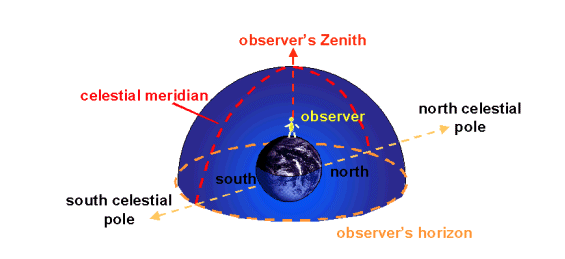https://astronomy.swin.edu.au/cosmos/c/Celestial+Meridian

The tilt of the orbit also has an effect on how long the moon is visible. Most readers will know that days are longer in the summer; that is that the sun is visible for more than 12 hours in a day. In the same way, the moon's visibility will change based on where it is in its orbit.

In the charts that follow, look at the relationship between moonrise and moonset and low tides.

Key West

In Key West, on Jan 5, 2023, the moon rose at 4:48 p.m. and was directly overhead at 11:57 p.m. (just before midnight). See the moon event table above. The moon, a little more than 12 hours later, was directly underfoot at 12:23 p.m. and set at 5.41 p.m.

The related high tides were at 8:35 p.m. (≈3.5 hours before the moon was over head) and at 10:15 a.m. (≈2.25 hours before it was underfoot).

------

In Honolulu, we saw this:

On Jan 5, 2023, the moon rose at 5:12 p.m. and was, on Jan 6, directly overhead at 12:13 a.m. (just after midnight). The moon, a little more than 12 hours later, was directly underfoot at 12:39 p.m. and set at 6:05 p.m.

High tides, however, occurred at 3:36 a.m. and at 3:06 p.m. (both ≈ 2.5 hours after the moon was overhead or under foot).

In the Honolulu table directly above, We can see that the timing of moonrise and low tide is far from the same. Clearly, more than just the moon affects tides.